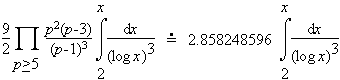# prime triple

A prime triple is three consecutive primes, such that the first and the last differ by six. For example:

(5, 7, 11), (7, 11, 13), (11, 13, 17), (13, 17, 19), (17, 19, 23), (37, 41, 43), (41, 43, 47), (67, 71, 73), (97, 101, 103), and (101, 103, 107).

It is conjectured that there are infinitely many such primes. In fact the Hardy-Littlewood prime k-tuple conjecture suggests that the number less than x of each of the forms

• (p, p+2, p+6) and
• (p, p+4, p+6)

is approximatelyThe actual numbers less than 100,000,000 are 55,600 and 55,556 respectively. The Hardy-Littlewood estimate above is 55,490.